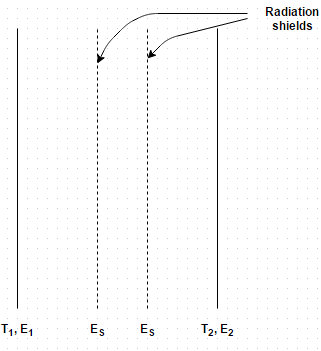# Test: Radiations Shields

Test Description

## 10 Questions MCQ Test Heat Transfer | Test: Radiations Shields

Test: Radiations Shields for Chemical Engineering 2022 is part of Heat Transfer preparation. The Test: Radiations Shields questions and answers have been prepared according to the Chemical Engineering exam syllabus.The Test: Radiations Shields MCQs are made for Chemical Engineering 2022 Exam. Find important definitions, questions, notes, meanings, examples, exercises, MCQs and online tests for Test: Radiations Shields below.
Solutions of Test: Radiations Shields questions in English are available as part of our Heat Transfer for Chemical Engineering & Test: Radiations Shields solutions in Hindi for Heat Transfer course. Download more important topics, notes, lectures and mock test series for Chemical Engineering Exam by signing up for free. Attempt Test: Radiations Shields | 10 questions in 10 minutes | Mock test for Chemical Engineering preparation | Free important questions MCQ to study Heat Transfer for Chemical Engineering Exam | Download free PDF with solutions
 1 Crore+ students have signed up on EduRev. Have you?
Test: Radiations Shields - Question 1

### A radiation shield should

Detailed Solution for Test: Radiations Shields - Question 1

Reflexive power is much high for radiation shield.

Test: Radiations Shields - Question 2

### Radiation shield are used between the emitting surfaces such that

Detailed Solution for Test: Radiations Shields - Question 2

Many situations are encountered where it is desired to reduce the overall heat transfer between two radiating surfaces.

Test: Radiations Shields - Question 3

### Which of the following can be used as a radiating shield?

Detailed Solution for Test: Radiations Shields - Question 3

The shields are thin opaque partitions arranged in the direction perpendicular to the propagation of radiant heat.

Test: Radiations Shields - Question 4

Two large parallel planes with emissivity 0.4 are maintained at different temperatures and exchange heat only by radiation. What percentage change in net radiative heat transfer would occur if two equally large radiation shields with surface emissivity 0.04 are introduced in parallel to the plates?Detailed Solution for Test: Radiations Shields - Question 4

When shields are not used, Q 12 = (F g) 12 A 1 σ b (T 14 – T 24) = 0.2 A 1 σ b (T 14– T 24) and when shields are used Q 12 = 0.0098 A 1 σ b (T 14 – T 24).

Test: Radiations Shields - Question 5

Determine the net radiant heat exchange per m 2 area for two infinite parallel plates held at temperature of 800 K and 500 K. Take emissivity as 0.6 for the hot plate and 0.4 for the cold plate

Detailed Solution for Test: Radiations Shields - Question 5

12 = (F g) 12 A 1 σ b (T 14 – T 24) and (F g) 12 = 0.135. Therefore, Q 12 = 6200 W/m2.

Test: Radiations Shields - Question 6

Consider the above problem, what should be the emissivity of a polished aluminum shield placed between them if heat flow is to be reduced to 40 percent of its original value?

Detailed Solution for Test: Radiations Shields - Question 6

(Fg) 12 = 1/E 1 +1/E 2 +2/E 3 – 2 = 7.936.

Test: Radiations Shields - Question 7

Consider radiative heat transfer between two large parallel planes of surface emissivities 0.8. How many thin radiation shields of emissivity 0.05 be placed between the surfaces is to reduce the radiation heat transfer by a factor of 75?

Detailed Solution for Test: Radiations Shields - Question 7

(Q 12ONE SHIELD = A σ b (T 14 – T 24)/ 1/E 1 +1/E 2 +2/E 3 – 2 and 75 = (Q 12NO SHIELD / (Q 12N SHIELD.

Test: Radiations Shields - Question 8

Two parallel square plates, each 4 m2 area, are large compared to a gap of 5 mm separating them. One plate has a temperature of 800 K and surface emissivity of 0.6, while the other has a temperature of 300 K and surface emissivity of 0.9. Find the net energy exchange by radiations between the plates

Detailed Solution for Test: Radiations Shields - Question 8

12 = (F g) 12 A 1 σ b (T 14 – T 24).

Test: Radiations Shields - Question 9

The furnace of a boiler is laid from fire clay brick with outside lagging from the plate steel, the distance between the two is quite small compared with the size of the furnace. The brick setting is at an average temperature of 365 K whilst the steel lagging is at 290 K. Calculate the radiant heat flux. Assume the following emissivity values
For brick = 0.85
For steel = 0.65

Detailed Solution for Test: Radiations Shields - Question 9

12 = (F g) 12 A 1 σ b (T 14 – T 24).

Test: Radiations Shields - Question 10

Consider the above problem, find the reduction in heat loss if a steel screen having an emissivity value of 0.6 on both sides is placed between the brick and steel setting

Detailed Solution for Test: Radiations Shields - Question 10

(F g) 12 = 0.247 and Q = 149.51 W/m2.

## Heat Transfer

58 videos|70 docs|85 tests
 Use Code STAYHOME200 and get INR 200 additional OFF Use Coupon Code